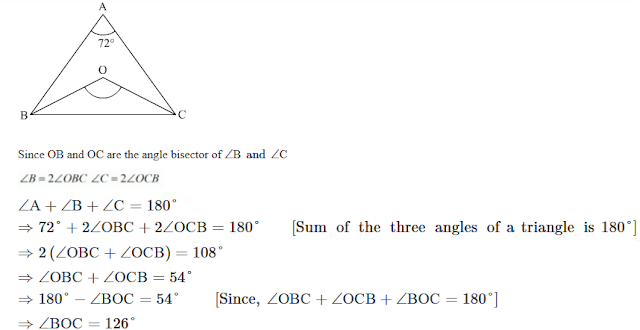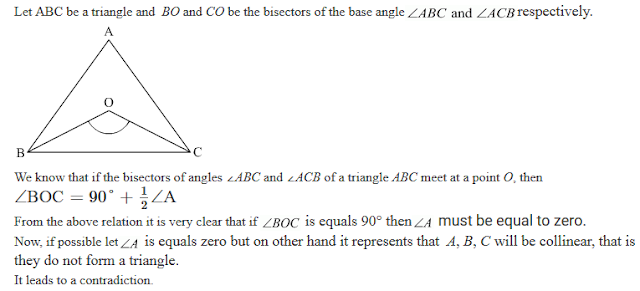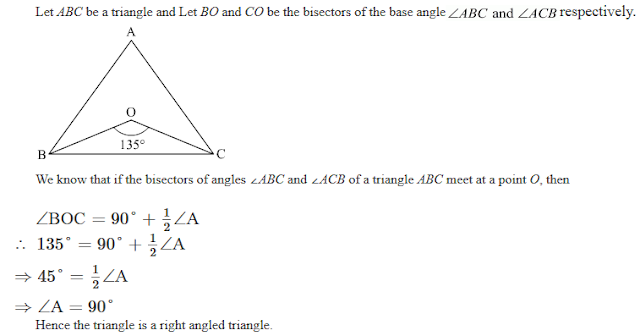#### Chapter 9 Triangle and its Angles R.D. Sharma Solutions for Class 9th Exercise 9.1

Exercise 9.1

1. In a Δ ABC, if ∠A = 55°, ∠B = 40°, find ∠C.

Solution2. If the angles of a triangle are in the ratio 1:2:3, determine three angles.

Solution

Let the angles of the given triangle be of x°, 2x° and 3x°. Then,
∴ x+2x+3x = 180° (The sum of three angles of a triangle is 180°)
⇒ 6x = 180°
⇒ x = 30°

3. The angles of a triangle are (x-40)°, (x-20 and (1/2x -10)°. Find the value of x.

Solution4. The angles of a triangle are arranged in ascending order of magnitude. If the difference between two consecutive angles is 10°, find the three angles.

Solution

Let the angles of a triangle x°, (x+10)° and (x+20)°.
Since, the difference between two consecutive angles is 10°.
∴ x+(x+10)+(x+20) = 180  (Sum of the three angles of a triangle is 180°.
⇒ 3x+30 = 180
⇒ 3x = 150
⇒ x = 50
Therefore, the angles of the given triangle are 50°, (50+10)° and (50+20)° i.e. 50°, 60° and 70°.

5. Two angles of a triangle are equal and the third angle is greater than each of those angles by 30°. Determine all the angles of the triangle.

Solution

Let the two equal angles are x°, then the third angle will be (x+30)°.
∴ x+x+(x+30) = 180  (Sum of the three angles of a triangle is 180°.)
⇒ 3x+30 = 180
⇒ 3x = 150
⇒ x = 50
Therefore, the angles of the given triangle are 50°, 50° and 80°.

6. If one angle of a triangle is equal to the sum of the other two, show that the triangle is a right triangle.

Solution

Let ABC be a triangle such that,
∠A = ∠B + ∠C  (Since, one angle is sum of the other two angles.)
∴ ∠A + (∠B +∠C) = 180  (Sum of the three angles of a triangle is 180°.)
⇒ ∠A + ∠A = 180
⇒ 2∠A = 180
⇒ ∠A = 90
Hence, the given triangle is a right angled triangle.

7. ABC is a triangle in which ∠A = 72°, the internal bisectors of angles B and C meet in O. Find the magnitude of ∠BOC.

Solution8. The bisectors of base angles of a triangle cannot enclose a right angle in any case.

Solution
Let ABC be a triangle and BO and CO be the bisectors of the base angle ∠ABC and ∠ACB respectively.9. If the bisectors of the base angles of a triangle enclose an angle of 135°, prove that the triangle is a right triangle.

Solution10. In a Δ ABC, ∠ABC = ∠ACB and the bisectors of ∠ABC and ∠ACB intersect at O such that ∠BOC = 120°. Show that ∠A =∠B =∠C = 60°.

Solution11. Can a triangle have:
(i) Two right angles?
(ii) Two obtuse angles?
(iii) Two acute angles?
(iv) All angles more than 60°?
(v) All angles less than 60°?
(vi) All angles equal to 60°?

Solution

(i) Let a triangle ABC has two angles ∠B and ∠C equal to 90. We know that sum of the three angles of a triangle is 180°.

∠A+∠B+∠C = 180°
⇒ 90° + 90° + ∠C = 180°  [∠A = 90° and ∠B = 90°]
⇒ 180 + ∠C = 180
⇒ ∠C = 0°
Hence, if two angles are equal to 90°, then the third one will be equal to zero which implies that A, B, C is collinear, or we can say ABC is not a triangle A triangle can’t have two right angles.

(ii) Let a triangle ABC has two obtuse angles  ∠B and ∠C.
This implies that sum of only two angles will be equal to more than 180° which contradicts the theorem sum of all angles in a triangle is always equals 180°.
Therefore, a triangle can’t have two obtuse angles.

(iii) Let a triangle ABC has two acute angles ∠B and ∠C.
This implies that sum of two angles will be less than 180°. Hence third angle will be the difference of 180° and sum of both acute angles
Therefore, a triangle can have two acute angles.

(iv) Let a triangle ABC having angles ∠A, ∠B and ∠C are more than 60°.
This implies that the sum of three angles will be more than 180° which contradicts the theorem sum of all angles in a triangle is always equals 180°.
Therefore, a triangle can’t have all angles more than 60°.

(v) Let a triangle ABC having angles ∠A, ∠B and ∠C are less than 60°.
This implies that the sum of three angles will be less than 180° which contradicts the theorem sum of all angles in a triangle is always equals 180°.
Therefore, a triangle can’t have all angles less than 60°.

(vi) Let a triangle ABC having angles ∠A, ∠B and ∠C all equal to 60°.
This implies that the sum of three angles will be equal to 180° which satisfies the theorem sum of all angles in a triangle is always equals 180°.
Therefore, a triangle can have all angles equal to 60°.

12. If each angle of a triangle is less than the sum of the other two, show that the triangle is acute angled.

Solution